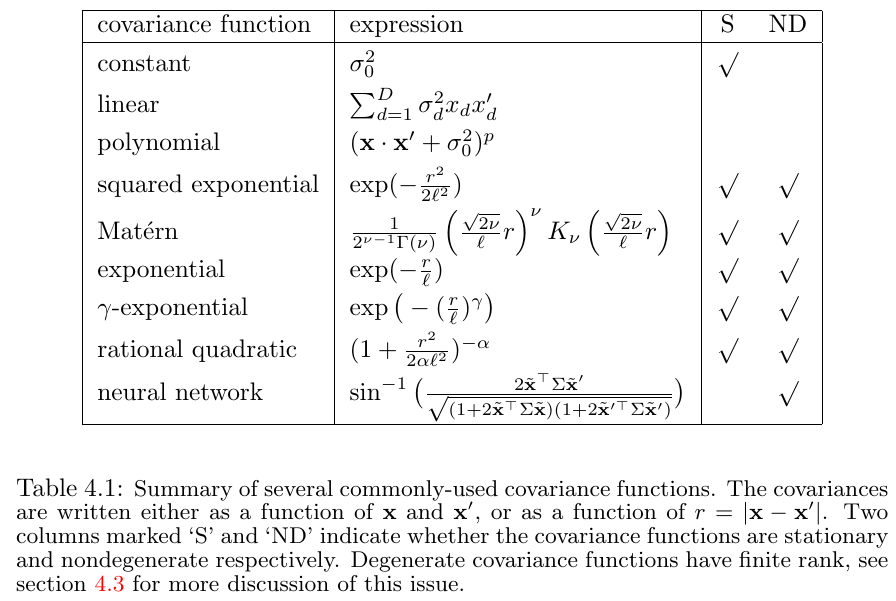# Gaussian Processes for Regression

##### Posted on Dec 13, 2021
Tags: Gaussian Processes

This note is for Chapter 4 of Rasmussen, C. E., & Williams, C. K. I. (2006). Gaussian processes for machine learning. MIT Press.

Dataset $\cD$ of $n$ observations, $\cD=\{(\x_i,y_i)\mid i=1,\ldots,n\}$

move from the finite training data $\cD$ to a function $f$ that makes predictions for all possible input values.

Two common approaches:

• restrict the class of functions, such as only considering linear functions of the input
• give a prior probability to every possible function, where higher probabilities are given to functions that we consider to be more likely, for example because they are smoother than other functions.

A Gaussian process is a generalization of the Gaussian probability distribution.

Several ways to interpret Gaussian process (GP) regression models.

• function-space view: think of a GP as defining a distribution over functions
• weight-space view:

## Weight-space view

### The Standard Linear Model

$f(x) = x^Tw, \qquad y=f(x) + \varepsilon, \qquad \varepsilon \sim N(0, \sigma_n^2)$

Put a zero mean Gaussian prior with covariance matrix $\Sigma_p$ on the weights

$w\sim N(0, \Sigma_p)$

we can obtain

$p(w\mid X, y) \sim N(\bar w, A^{-1})$

where the mean is also its mode, which is also called the maximum a posterior (MAP) estimate of $w$.

In a non-Bayesian setting, the negative log prior is sometimes thought of as a penalty term, and the MAP point is known as the penalized maximum likelihood estimate of the weights.

To make predictions for a test case, we average over all possible parameter values, weighted by their posterior probability. Thus the predictive distribution for $f_\star$ at $x_\star$ is given by averaging the output of all possible linear models w.r.t. the Gaussian posterior,

$p(f_\star\mid x_\star,X,y) = \int p(f_\star\mid x_\star,w)p(w\mid X, y)dw$

### Projections of Inputs into Feature Space

Introduce the function $\phi(x)$ which maps a D-dimensional input vector $x$ into an $N$ dimensional feature space.

$f(x) = \phi(x)^Tw$

Define $k(x,x’)=\phi(x)’\Sigma_p\phi(x’)$ as a covariance function or kernel.

kernel trick: if an algorithm is defined solely in terms of inner products in input space then it can be lifted into feature space by replacing occurrences of those inner products by $k(x,x’)$.

## Function-space View

A Gaussian process is a collection of random variables, any finite number of which have a joint Gaussian distribution.

A GP is completely specified by its mean function and covariance function,

$f(x) \sim GP(m(x), k(x, x'))$

marginalization property: if the GP specifies $(y_1,y_2)\sim N(\mu,\Sigma)$, then it must also specify $y_1\sim N(\mu_1,\Sigma_{11})$

squared exponential (SE) covariance function:

$\cov(f(x_p), f(x_q)) = k(x_p,x_q) = \exp(-\frac 12\vert x_p-x_q\vert^2)$

The specification of the covariance function implies a distribution over functions. We can draw samples from the distribution of functions evaluated at any number of points.

## Varying the Hyperparameters

The SE covariance function in one dimension has the following form

$k_y(x_p, x_q) = \sigma_f^2 \exp\left(-\frac{1}{2\ell^2}(x_p-x_q)^2\right) + \sigma^2_n\delta_{pq}$

Other kernel functions,### The Matérn Class of Covariance Functions

• $\nv \rightarrow\infty$, it becomes the SE kernel
• it becomes simple when $\nv$ is half-integer: $\nv = p+1/2$. In this case, the covariance function is a product of an exponential and a polynomial of order $p$
• for $\nv \ge 7/2$, in the absence of explicit prior knowledge about the existence of higher order derivatives, it is probably very hard from finite noisy training examples to distinguish between values of $\nv \ge 7/2$ (or even to distinguish between finite values of $\nv$ and $\nv\rightarrow\infty$)

### Rational Quadratic Covariance Function

It can be seen as a scale mixture (an infinite sum) of squared exponential (SE) covariance functions with different characteristic length-scales

if $\alpha\rightarrow\infty$, the limit of the RQ covariance is the SE covariance function

Published in categories Note